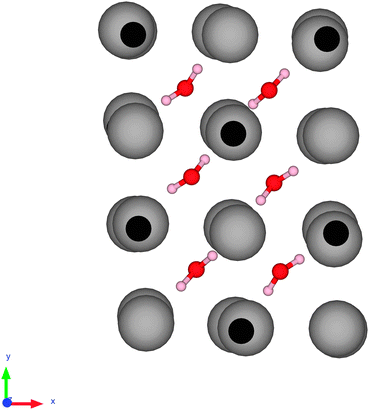# Question #2cd42

Jan 9, 2017

Here's my understanding.

#### Explanation:

Coordinated water is water that is a ligand in a metal complex.

The metal atom and the water molecules are held together by coordinate bonds, as in $\text{Cu"("H"_2"O")_6^"2+}$Hydrogen-bonded water is the water that that is linked to some other ion by hydrogen bonding.

For example, solid $\text{CuSO"_4·5"H"_2"O}$ is better represented as $\text{Cu"("H"_2"O")_4^"2+" "SO"_4^"2-"·"H"_2"O}$.

The $\text{Cu"^"2+}$ ion is coordinated to four water molecules in a square planar geometry, with the sulfate ions in the axial positions.

The fifth water molecule is hydrogen bonded to the sulfate ion.

That means the two water molecules on the axial positions of $\text{Cu"("H"_2"O")_6^"2+}$ are eliminated and substituted by $\text{SO"_4^"2-}$ ions when the solid is formed.Interstitial water is water that is present in the interstitial sites of a crystal lattice, as in hydrated $\text{RbBr}$

In the diagram below, the small dark spheres are $\text{Br}$ atoms, the large grey spheres are $\text{Rb}$, and water molecules occupy the interstitial cavities of the crystal.